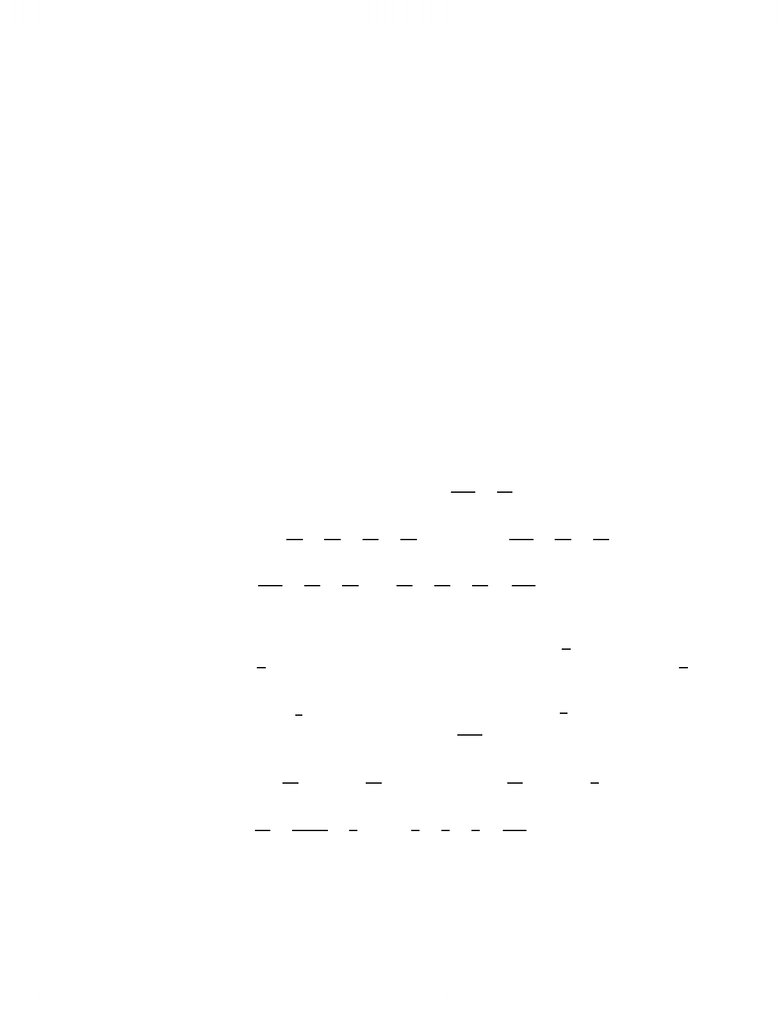# MATA33H3 Lecture Notes - Multiple Integral, Farad, Elementary Function

33 views3 pages
School
UTSC
Department
Mathematics
Course
MATA33H3
ProfessorReversing the Order of Integration
Let z=f(x, y) and let Bbe a subset of the Xy-plane. The volume of the ﬁgure
lying below the graph of f(x, y) and over the set Bis denoted RBf(x, y)dA To compute
it requires a double integral. If Bis the region between the curves y=g(x) (below) and
y=h(x) (above) for xbetween aand b, then RBf(x, y)dA =Rb
aRh(x)
g(x)f(x, y)dy dx. Note
that the limits of integration depend only the region Band are independent of the function
z=f(x, y) being integrated. Since the preceding discussion is symmetrical with respect to
xand y, we cab reverse the roles of xand yif convenient. That is, if Bis written instead
as the region bounded by the curves x=p(y) (on the left) and x=q(y) (on the right) for y
between cand dthen RBf(x, y)dA is also given by RBf(x, y)dA =Rd
cRq(y)
p(y)f(x, y)dx dy.
Example. Let f(x, y) = xy +y2and let Bbe the region between the curves y=x
and y=x2. Compute RBf(x, y)dA.
Solution 1.
The curves y=xand y=x2intersect at x= 0 and x= 1. For xbetween 0 and 1,
x2x. Therefore Bis the region between y=x2(below) and y=x(above) for xbetween
0 and 1. Therefore
ZB
f(x, y)dA =Z1
0Z2
x
(xy +y2)dy dx =Z1
0xy2
2+y3
3y=x
y=x2
dx
=Z1
0x3
2+x3
3x5
2x6
3dx =Z1
05x3
6x5
2x6
3dx
=5x4
24 x6
12 x7
211
0
=5
24 1
12 1
21 =13
168
Solution 2.
Solving for the curves for xin terms of ygives x=yand x=yrespectively. The
curves x=yand x=yintersect at y= 0 and y= 1. For ybetween 0 and 1, yy.
Therefore
ZB
f(x, y)dA =Z1
0Zy
y
(xy +y2)dx dy =Z1
0x2y
2+xy2x=y
x=y
dy
=Z1
0y2
2+y5/2y3
2y3dy =Z1
0y2
2+y5/23
2y3dy
=y3
62y7/2
73
6y41
0
=1
62
73
8=13
168
as before. Note that the fact that the outer limits came out to be 0 and 1 after reversing
the order, just as they were before, is a coincidence: the curves meet at the points (0,0)
and (1,1) which happen to have the same xand ycoordinates.
1
Unlock document

This preview shows page 1 of the document.
Unlock all 3 pages and 3 million more documents.